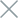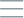• Home
• Tags
• Series
•# Python Functions: Definition, Parameters and Return Values

## Function and Defining Functions in Python

In Python, a function is a block of code that performs a specific task and can be reused throughout the program. Defining a function in Python involves the following steps:

## Function Definition Syntax

To define a function in Python, you use the `def` keyword, followed by the function name and a list of input parameters enclosed in parentheses `()`. The code that performs the function's task is placed inside the function's body, which is indented inside the `def` block. A function can return a value (or multiple values) using the `return` keyword. If there is no `return` statement in the function, the function will automatically return `None`.

## Using Input Parameters

A function can receive information from the outside through input parameters. Parameters are the values that you provide when calling the function. These parameters will be used within the function's body to perform specific tasks.

## Returning Values from a Function

Once the function has completed its task, you can use the `return` keyword to return a value from the function. If the function does not have a `return` statement, the function will automatically return `None`.

## Calling a Function

To use a defined function, you simply call the function's name and pass any required parameter values (if any). The result returned from the function (if any) can be stored in a variable for future use or printed to the screen.

## Detailed Example

``````# Define a function to calculate the sum of two numbers
def calculate_sum(a, b):
sum_result = a + b
return sum_result

# Define a function to greet the user
def greet_user(name):
return "Welcome, " + name + "!"

# Call the functions and print the results
num1 = 5
num2 = 3
result = calculate_sum(num1, num2)
print("The sum of", num1, "and", num2, "is:", result)  # Output: The sum of 5 and 3 is: 8

name = "John"
greeting_message = greet_user(name)
print(greeting_message)  # Output: Welcome, John!
``````

In the example above, we have defined two functions: `calculate_sum()` to calculate the sum of two numbers and `greet_user()` to create a greeting message. Then, we called these functions and printed the results.